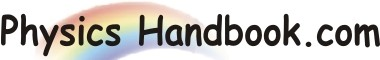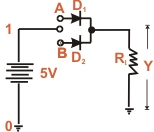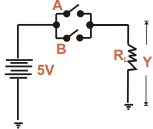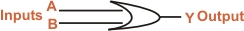HOME TOPICS DEFINITIONS TABLES LAWS INVENTIONS EXPERIMENTS QUIZ VIDEOS
 A B C D E F G H I J K L M N O P Q R S T U V W X Y Z
OR Gate
An OR gate is a logic gate that has two or more inputs but only one output. However, the output Y of an OR gates is LOW when all inputs are LOW. The output Y of an OR gate is HIGH if any or all the inputs are HIGH.
It is called OR gate because the output is high if any or all the inputs are high. For the sane reason, an OR gate is sometimes called "any or all gate". For example, consider a 2-input OR gate. The output y will be high if either or both input are high.Fig (a) Fig (b)
OR gate Operation : Fig (a) shown one way to build a 2-inputs OR gate while Fig (b) shows its simplified schematic diagram. The input voltage are labeled as A and B corresponds to 0 state (LOW level). The positive terminal of the battery (+5V) corresponds to 1 state HIGH level). There are only four input-output possibilities.

(I) When both A and B are connected to ground, both diodes are non-conducting. Hence the out put voltage is ideally zero (lo voltage). In terms of binary, when A = 0 and B = 0, then Y = 0 as shows in the truth table.

(II) When A is connected to ground and B connected to the positive terminal of the battery, diode D2 is forward biased and diode D1 is non-conducting. Therefore diode D2 conducts and the output voltage is ideally +5V. In terms of binary, When A = 0 and B = 1,then Y = 1 as shows in the truth table.

(III) When both A and B are connected to the positive terminal of the battery and B to the ground, diode D1 is on and diode D2 is off. Again the output voltage is +5V. In binary terms when A = 1 and B = 0 then Y = 1 as shows in the truth table.

(IV) When both A and B are connected to the positive terminal of the battery, both diodes are on. Since the diodes are in parallel, the output voltage is +5V. In binary terms, when A = 1 and B = 1 ,then Y = 1 as shows in the truth table.

A
B
Y

0
0
0
0
1
1
1
0
1
1
1
1
Truth Table
IT is clear from the truth table that for OR gate, the output is high if any or all of the inputs are high. The only way to get a low output is by having all inputs low. Fig (C) shows the logical symbol of OR gate. Note that the symbol has curved line at the inputFig (c)
Boolean Expression : The algebra used to symbolically describe logic function is called Boolean algebra. The "+" sign in Boolean algebra refers to the logical OR function. The Boolean expression for OR function is
A + B = Y where "+" is OR symbol
A + B
Y

0 + 0
0
0 + 1
1
1 + 0
1
1 + 1
1